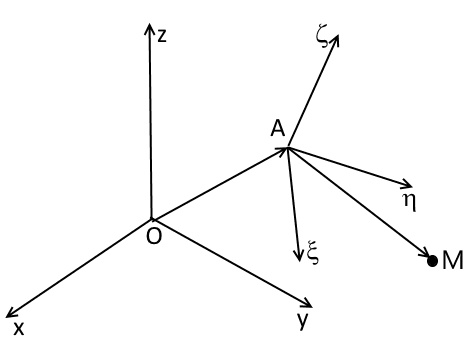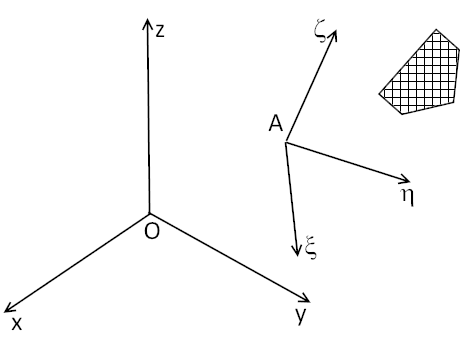# Basic Kinematics in Classical Mechanics

[Total: 2    Average: 5/5]

There is an interesting thing in teaching of Classical Mechanics. Several theorems which presented below form a core part of kinematics for all Russian textbooks in Classical Mechanics (excluding Classical Mechanics for physicists)  but European and USA textbooks contain this material just partly and from time to time. Perhaps I just do not know enough about western and American style of teaching of this subject. It would be very interesting for me to hear comments about teaching of this part of the course of Classical Mech in USA and EU.

Assume  we have a fixed coordinate frame ##Oxyz##,  a moving frame ##A\xi\eta\zeta## and a point ##M## which moves in accordance to a law of motion $$\boldsymbol {OM}=\boldsymbol {OM}(t)=x(t)\boldsymbol e_x+y(t)\boldsymbol e_y+z(t)\boldsymbol e_z.$$Velocity and acceleration of ##M## obviously are $$\boldsymbol v^M=\dot x(t)\boldsymbol e_x+\dot y(t)\boldsymbol e_y+\dot z(t)\boldsymbol e_z,\quad \boldsymbol a^M=\ddot x(t)\boldsymbol e_x+\ddot y(t)\boldsymbol e_y+\ddot z(t)\boldsymbol e_z.$$

If ##\boldsymbol {AM}(t)=\xi(t)\boldsymbol e_\xi+\eta(t)\boldsymbol e_\eta+\zeta(t)\boldsymbol e_\zeta## then by definition the velocity and acceleration of ##M## relative to the frame ##A\xi\eta\zeta## are given by the formulas

$$\boldsymbol v_r^M=\dot \xi(t)\boldsymbol e_\xi+\dot\eta(t)\boldsymbol e_\eta+\dot\zeta(t)\boldsymbol e_\zeta,\quad \boldsymbol a_r^M=\ddot \xi(t)\boldsymbol e_\xi+\ddot \eta(t)\boldsymbol e_\eta+\ddot \zeta(t)\boldsymbol e_\zeta.$$

Definition. Let us imagine that the point ##M## is attached rigidly to the moving frame ##A\xi\eta\zeta## i.e. its coordinates ##\xi,\eta,\zeta## do not change. Under this assumption the point ##M## is connected with the moving frame would possess a certain velocity and a certain acceleration relative to the fixed frame. These velocity and acceleration are called the velocity of transport and the acceleration of transport and denoted by ##\boldsymbol v_t^M,\boldsymbol a_t^M## respectively.

Let ##\boldsymbol \omega_t## stand for the angular velocity of the frame ##A\xi\eta\zeta## and ##\boldsymbol \epsilon_t=\dot{\boldsymbol\omega}_t## be the angular acceleration of the frame ##A\xi\eta\zeta##.

Definition. The vector ##\boldsymbol a_c^M=2\boldsymbol\omega_t\times \boldsymbol v_r^M## is called the Coriolis acceleration.

Theorem. The following formulas hold

$$\boldsymbol v^M=\boldsymbol v_r^M+\boldsymbol v_t^M,\quad \boldsymbol a^M=\boldsymbol a_r^M+\boldsymbol a_t^M+\boldsymbol a_c^M;$$ and

$$\boldsymbol v_t^M=\boldsymbol v^A+\boldsymbol\omega_t\times\boldsymbol{AM},\quad \boldsymbol a_t^M=\boldsymbol a^A+\boldsymbol \epsilon_t\times \boldsymbol{AM}+\boldsymbol\omega_t\times(\boldsymbol\omega_t\times\boldsymbol{AM}).$$

Now assume that we have a moving rigid body and its angular velocity and angular acceleration are ##\boldsymbol \omega,\boldsymbol\epsilon.## Let  ##\boldsymbol \omega_r,\boldsymbol\epsilon_r## stand for angular velocity and angular acceleration of the rigid body relative to the frame ##A\xi\eta\zeta##.Theroem. The following formulas hold

$$\boldsymbol \omega=\boldsymbol \omega_t+\boldsymbol \omega_r,\quad \boldsymbol \epsilon=\boldsymbol \epsilon_t+\boldsymbol \epsilon_r+\boldsymbol \omega_t\times\boldsymbol \omega_r.$$

Tags:
7 replies
1.vanhees71 says:

Here in Germany this is usually taught in the theory course (at Goethe University Frankfurt even already in the 1st semester!) in the context of rigid-body motion (basic theory of the spinning top).

2.wrobel says:

Thanks. Do know textbooks in English that contain this material?

3.wrobel says:

in these fromulas it is important that the moving frame moves in arbitrary way it can rotate for example

4.vanhees71 says:

E.g., you find it in

Kibble, Berkshire, Classical Mechanics, Imperial College Press

I guess, in fact, you find it in any university theoretical-physics textbook.

5.wrobel says:

O now I see how it is taught in the western tradition

6.Thanks @wrobel!

7.ZapperZ says:

I think that the title is a bit misleading. This is not "basic kinematics". For most people, or at least for me, basic kinematics are the kinematical equations that intro physics students see for motion with constant acceleration.

This article, which include rigid body motion and coriolis force, is way more advanced than those kinematical equations.

Zz.

8.fog37 says:

Hello, I have tried to expand my horizon on "basic" kinematics and I run into the concept of Mozzi axis of instantaneous rotation. Have you heard about it before?

If so, I have some follow up questions. Thanks